Definition: Pascal's triangle from Collins English Dictionary

n

1 a triangle consisting of rows of numbers; the apex is 1 and each row starts and ends with 1, other numbers being obtained by adding together the two numbers on either side in the row above: used to calculate probabilities

[C17: named after Blaise Pascal]

Summary Article: Pascal's triangle
from 50 Maths Ideas You Really Need to Know

The number 1 is important but what about 11? It is interesting too and so is 11 × 11 = 121, 11 × 11 × 11 = 1331 and 11 × 11 × 11 × 11 = 14,641. Setting these out we get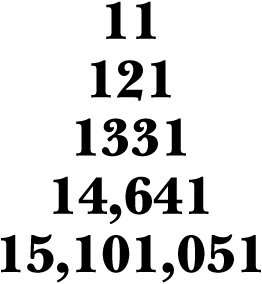These are the first lines of Pascal's triangle. But where do we find it?

Throwing in 10 = 1 for good measure, the first thing to do is forget the commas, and then introduce spaces between the numbers. So 14,641 becomes 1 4 6 4 1.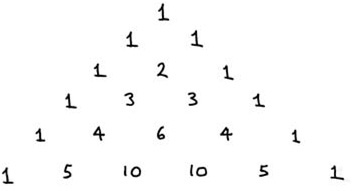Pascal's triangle

Pascal's triangle is famous in mathematics for its symmetry and hidden relationships. In 1653 Blaise Pascal thought so and remarked that he could not possibly cover them all in one paper. The many connections of Pascal's triangle with other branches of mathematics have made it into a venerable mathematical object, but its origins can be traced back much further than this. In fact Pascal didn't invent the triangle named after him – it was known to Chinese scholars of the 13th century.

The Pascal pattern is generated from the top. Start with a 1 and place two 1s on either side of it in the next row down. To construct further rows we continue to place 1s on the ends of each row while the internal numbers are obtained by the sum of the two numbers immediately above. To obtain 6 in the fifth row for example, we add 3 + 3 from the row above. The English mathematician G.H. Hardy said ‘a mathematician, like a painter or a poet, is a maker of patterns’ and Pascal's triangle has patterns in spades.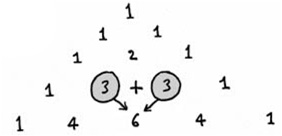Pascal's triangle is founded on real mathematics. If we work out (1 + x) × (1 + x) × (1 + x) = (1 + x)3, for example, we get 1 + 3x + 3x2 + x3. Look closely and you'll see the numbers in front of the symbols in this expression match the numbers in the corresponding row of Pascal's triangle. The scheme followed is: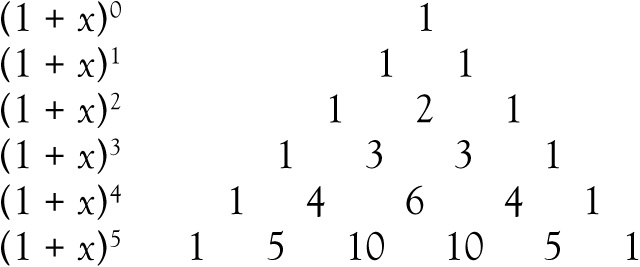If we add up the numbers in any row of Pascal's triangle we always obtain a power of 2. For example in the fifth row down 1 + 4 + 6 + 4 + 1 = 16 = 24. This can be obtained from the left-hand column above if we use x = 1.

Properties

The first and most obvious property of Pascal's triangle is its symmetry. If we draw a vertical line down through the middle, the triangle has ‘mirror symmetry’ – it is the same to the left of the vertical line as to the right of it. This allows us to talk about plain ‘diagonals’, because a northeast diagonal will be the same as a northwest diagonal. Under the diagonal made up of 1s we have the diagonal made up of the counting numbers 1, 2, 3, 4, 5, 6, … Under that there are the triangular numbers, 1, 3, 6, 10, 15, 21, … (the numbers which can be made up of dots in the form of triangles). In the diagonal under that we have the tetrahedral numbers, 1, 4, 10, 20, 35, 56, … These numbers correspond to tetrahedra (‘three-dimensional triangles’, or, if you like, the number of cannon balls which can be placed on triangular bases of increasing sizes). And what about the ‘almost diagonals’?

If we add up the numbers in lines across the triangle (which are not rows or true diagonals), we get the sequence 1, 2, 5, 13, 34, … Each number is three times the previous one with the one before that subtracted. For example 34 = 3 × 13 – 5. Based on this, the next number in the sequence will be 3 × 34 – 13 = 89. We have missed out the alternate ‘almost diagonals’, starting with 1, 1 + 2 = 3, but these will give us the sequence 1, 3, 8, 21, 55, … and these are generated by the same ‘3 times minus 1’ rule. We can therefore generate the next number in the sequence, as 3 × 55 – 21 = 144. But there's more. If we interleave these two sequences of ‘almost diagonals’ we get the Fibonacci numbers:

1, 1, 2, 3, 5, 8, 13, 21, 34, 55, 89, 144, …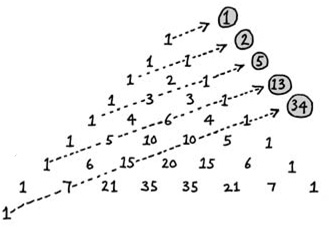Almost diagonals in Pascal's triangle

Pascal combinations

The Pascal numbers answer some counting problems. Think about 7 people in a room. Let's call them Alison, Catherine, Emma, Gary, John, Matthew and Thomas. How many ways are there of choosing different groupings of 3 of them? One way would be A, C, E; another would be A, C,T. Mathematicians find it useful to write C(n,r) to stand for the number in the nth row, in the rth position (counting from r = 0) of Pascal's triangle. The answer to our question is C(7,3). The number in the 7th row of the triangle, in the 3rd position, is 35. If we choose one group of 3 we have automatically selected an ‘unchosen’ group of 4 people. This accounts for the fact that C(7,4) = 35 too. In general, C(n,r) = C(n, nr) which follows from the mirror symmetry of Pascal's triangle.

0s and 1s

In Pascal's triangle, we see that the inner numbers form a pattern depending on whether they are even or odd.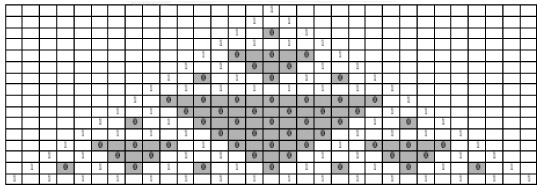Even and odd numbers in Pascal's triangle

If we substitute 1 for the odd numbers and 0 for the even numbers we get a representation which is the same pattern as the remarkable fractal known as the Sierpinski gasket (see Chapter 25).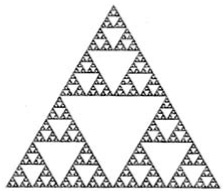We can write down the Pascal triangle that corresponds to the powers of (−1 + x), namely (−1 + x)n.

In this case the triangle is not completely symmetric about the vertical line, and instead of the rows adding to powers of 2, they add up to zero. However it is the diagonals which are interesting here. The southwestern diagonal 1, −1, 1, −1, 1, −1, 1, −1, … are the coefficients of the expansion while the terms in the next diagonal along are the coefficients of the expansion

(1 + x)−1 = 1 − x + x2 − x3 + x4 − x5 + x6 − x7 + …

(1 + x)−2 = 1 – 2x + 3x2 – 4x3 + 5x4 – 6x5 + 7x6 – 8x7 + …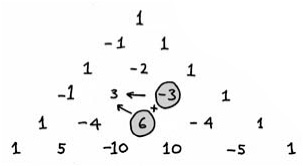The Leibniz harmonic triangle

The German polymath Gottfried Leibniz discovered a remarkable set of numbers in the form of a triangle. The Leibniz numbers have a symmetry relation about the vertical line. But unlike Pascal's triangle, the number in one row is obtained by adding the two numbers below it. For example 1/30 + 1/20 = 1/12.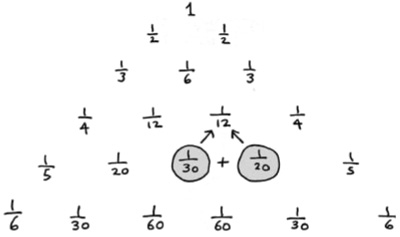The Leibniz harmonic triangle

To construct this triangle we can progress from the top and move from left to right by subtraction: we know 1/12 and 1/30 and so 1/121/30 = 1/20, the number next to 1/30. You might have spotted that the outside diagonal is the famous harmonic series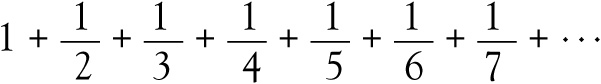but the second diagonal is what is known as the Leibnizian series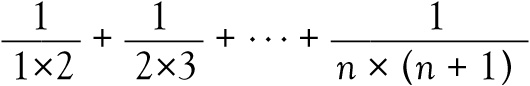which by some clever manipulation turns out to equal n/(n + 1). Just as we did before, we can write these Leibnizian numbers as B(n,r) to stand for the nth number in the rth row. They are related to the ordinary Pascal numbers C(n,r) by the formula: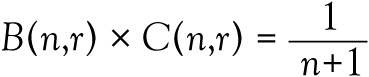In the words of the old song, ‘the knee bone's connected to the thigh bone, and the thigh bone's connected to the hip bone’. So it is with Pascal's triangle and its intimate connections with so many parts of mathematics – modern geometry, combinatorics and algebra to name but three. More than this it is an exemplar of the mathematical trade – the constant search for pattern and harmony which reinforces our understanding of the subject itself.

the condensed idea

The number fountain

Timeline
c.500 BC

Fragmentary evidence exists for Pascal's triangle in Sanscrit

Omar Khayyam discovers the triangle, which in some countries is named after him

1303

Zhu Shijie defines Pascal's triangle and shows how to sum certain sequences

1664

Pascal's paper on the properties of the triangle is published posthumously

1714

Leibniz discusses the harmonic triangleCopyright © Tony Crilly 2007

Related Articles

Full text Article Pascal's triangle
Dictionary of Eponyms

The French mathematician and philosopher Blaise Pascal ( 1623 - 1662 ) had an early interest in mathematics that led to his formulation of...

Full text Article Pascal's triangle
The Penguin Dictionary of Mathematics

A triangular arrangement of numbers as shown above. The numbers give the coefficients for the expansion of ( x + y) n . The first row is for n...

See more from Credo